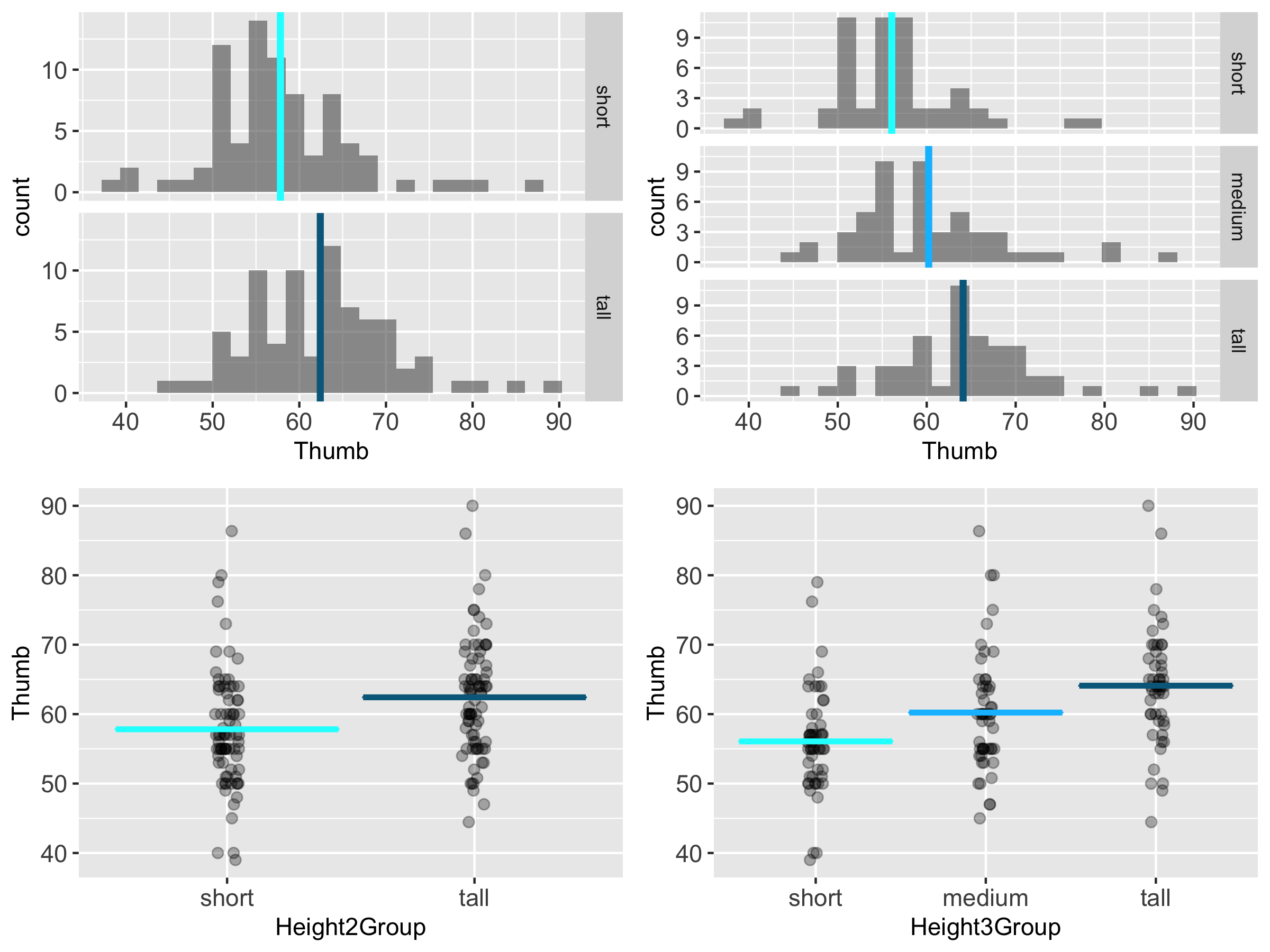## Course Outline

• segmentGetting Started (Don't Skip This Part)
• segmentStatistics and Data Science: A Modeling Approach
• segmentPART I: EXPLORING VARIATION
• segmentChapter 1 - Welcome to Statistics: A Modeling Approach
• segmentChapter 2 - Understanding Data
• segmentChapter 3 - Examining Distributions
• segmentChapter 4 - Explaining Variation
• segmentPART II: MODELING VARIATION
• segmentChapter 5 - A Simple Model
• segmentChapter 6 - Quantifying Error
• segmentChapter 7 - Adding an Explanatory Variable to the Model
• segmentChapter 8 - Models with a Quantitative Explanatory Variable
• segmentPART III: EVALUATING MODELS
• segmentChapter 9 - The Logic of Inference
• segmentChapter 10 - Model Comparison with F
• segmentChapter 11 - Parameter Estimation and Confidence Intervals
• segmentPART IV: MULTIVARIATE MODELS
• segmentChapter 12 - Introduction to Multivariate Models
• segmentChapter 13 - Multivariate Model Comparisons
• segmentFinishing Up (Don't Skip This Part!)
• segmentResources

### list College / Advanced Statistics and Data Science (ABCD)

Book
• High School / Statistics and Data Science I (AB)
• College / Statistics and Data Science (ABC)
• High School / Advanced Statistics and Data Science I (ABC)
• College / Advanced Statistics and Data Science (ABCD)
• High School / Statistics and Data Science II (XCD)

## 7.11 Improving Models by Adding Parameters

You probably noticed in the previous section that the three-parameter Height3Group model explained more variation than the Height2Group model; that is, it reduced the unexplained error more than the Height2Group model when compared with the empty model.

If we look at histograms and jitter plots for the two-group model and the three-group model (below) you can get a sense of why this is. By adding more categories for height we are able to reduce the error variation around the mean height for each group.In general, the more parameters we add to a model the less leftover error there is after subtracting out the model. Because we have said, many times, that the goal of the statistician is to reduce error, this seems like a good thing. And it is, but only to a point.

Let’s do a little thought experiment. You know already that the three-group model explained more variation than the two-group model. The four-group model would explain more than the three-group. And so on. What would happen if we kept splitting into more groups until each person was in their own group?

If each person were in their own group, the error would be reduced to 0. Why? Because each person would have their own parameter in the model. If each person had their own parameter, then the predicted score for that person would just be the person’s actual score. And there would be no residual between the predicted and actual score.

There are two problems with this. First, even though the model fits our data perfectly, it would not fit if we were to choose another sample. Second, the purpose of creating a more complex model is to help us understand the Data Generating Process. It’s okay to add a little complexity to a model if it leads to better understanding. But if we have as many parameters as we have people, we have added a lot of complexity without contributing to our understanding of the DGP.

Although we can improve model fit by adding parameters to a model, there is always a trade-off involved between reducing error (by adding more parameters to a model), on one hand, and increasing the intelligibility, simplicity, and elegance of a model, on the other.

This is a limitation of PRE as a measure of our success. If we get a PRE of .40, for example, that would be quite an accomplishment if we had only added a single parameter to the model. But if we had achieved that level by adding 10 parameters to the model, well, it’s just not as impressive. Statisticians sometimes call this “overfitting.”

There is a quote attributed to Einstein that sums up things pretty well: “Everything should be made as simple as possible, but not simpler.” A certain amount of complexity is required in our models just because of complexity in the world. But if we can simplify our model so as to help us make sense of complexity, and make predictions that are “good enough,” that is a good thing.